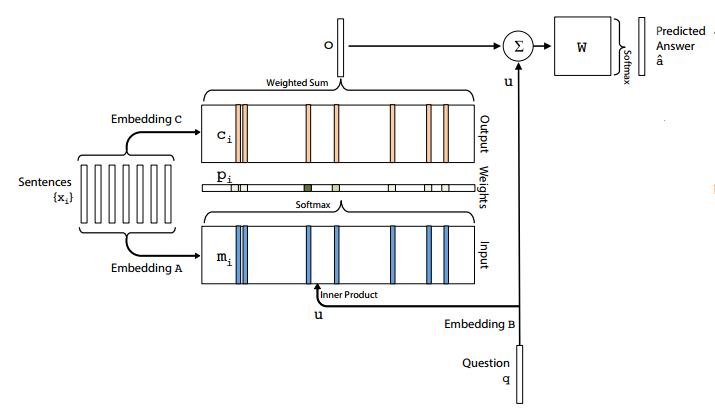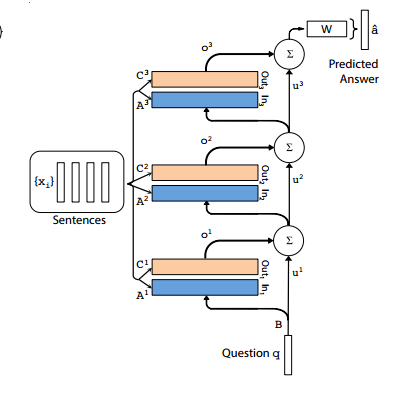# 论文笔记：End-to-End Memory Networks

1. Memory Networks 难以进行 BP 训练
2. Memory Networks 需要更多的 supervision(这个没太懂，回头把 Memory Networks 再看一下)$p=softmax(u^{T}M)$

$o=\sum_{i}p_{i}c_{i}$

$u^{k+1} = o^{k}+u^{k}$1. Adjacent: 将前一层的输出 embedding 矩阵 C 作为本层的输入 embedding 矩阵 A: $$A^{k+1}=C^{k}$$
2. Layer-wise: 像 RNN 一样，所有输入 embedding 矩阵 A 共享参数: $$A^{1}=A^{2}=...=A^{K}$$，输出矩阵 C 同理

1. BoW: 简单的将句子中每个词的 embedding 累加起来: $$m_{i}=\sum_{j}Ax_{ij}$$，u 和 $$c_{i}$$ 的计算同理
2. position encoding: $$m_{i}=\sum_{j}l_{j}\cdot Ax_{ij}$$，这里的 $$l_{j}$$ 就是所谓的反映了词序的 position encoding，它和后面的值进行 element-wise 的乘法而不是內积，而这个 position encoding 的计算方法是: $$l_{kj}=(1-j/J)-(k/d)(1-2j/J)$$，其中 j 是词在句子中的位置，J 是句子的长度，k 是这个词的 vocabulary id，d 是 vocabulary 的大小。

$m_{i}=\sum_{j}Axij + T_{A}(i)$